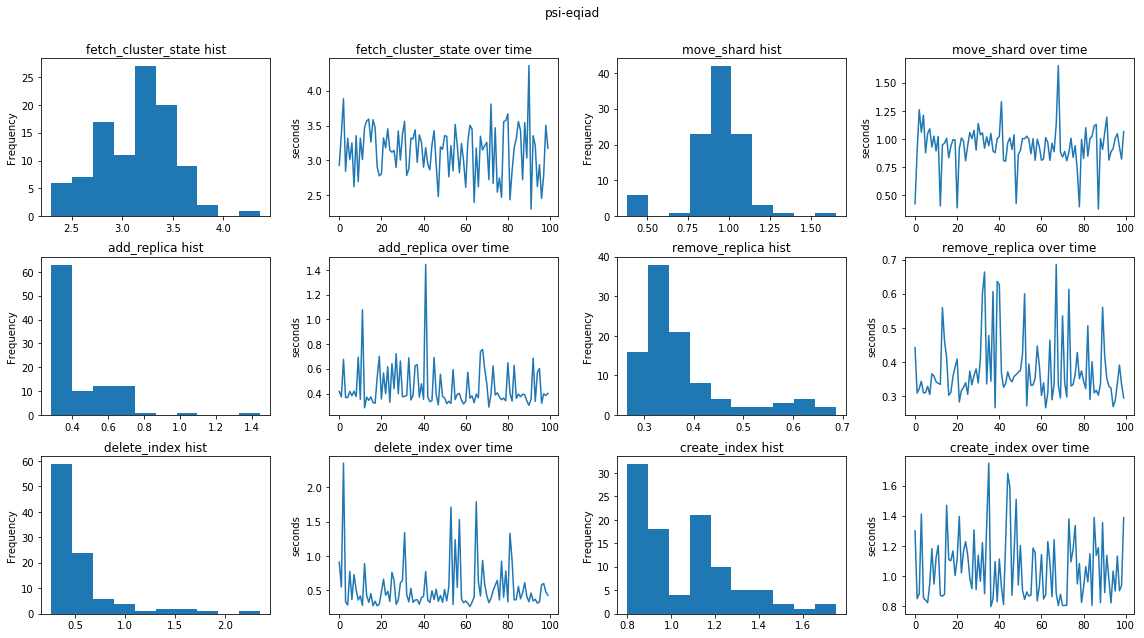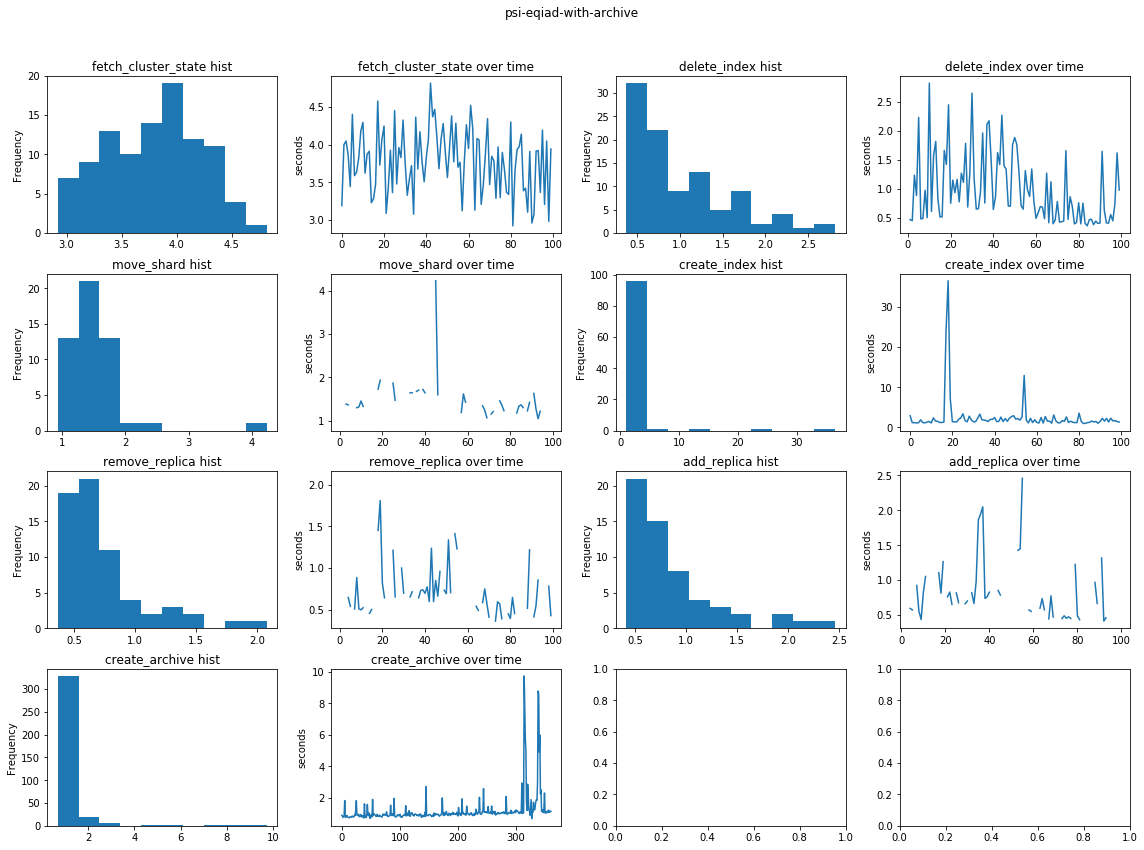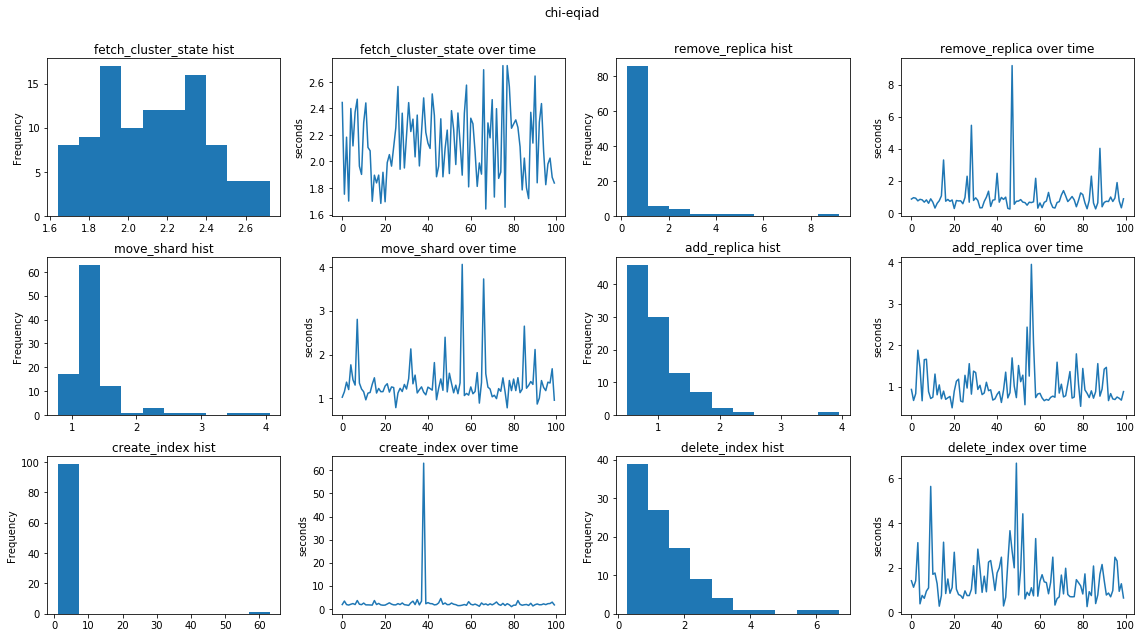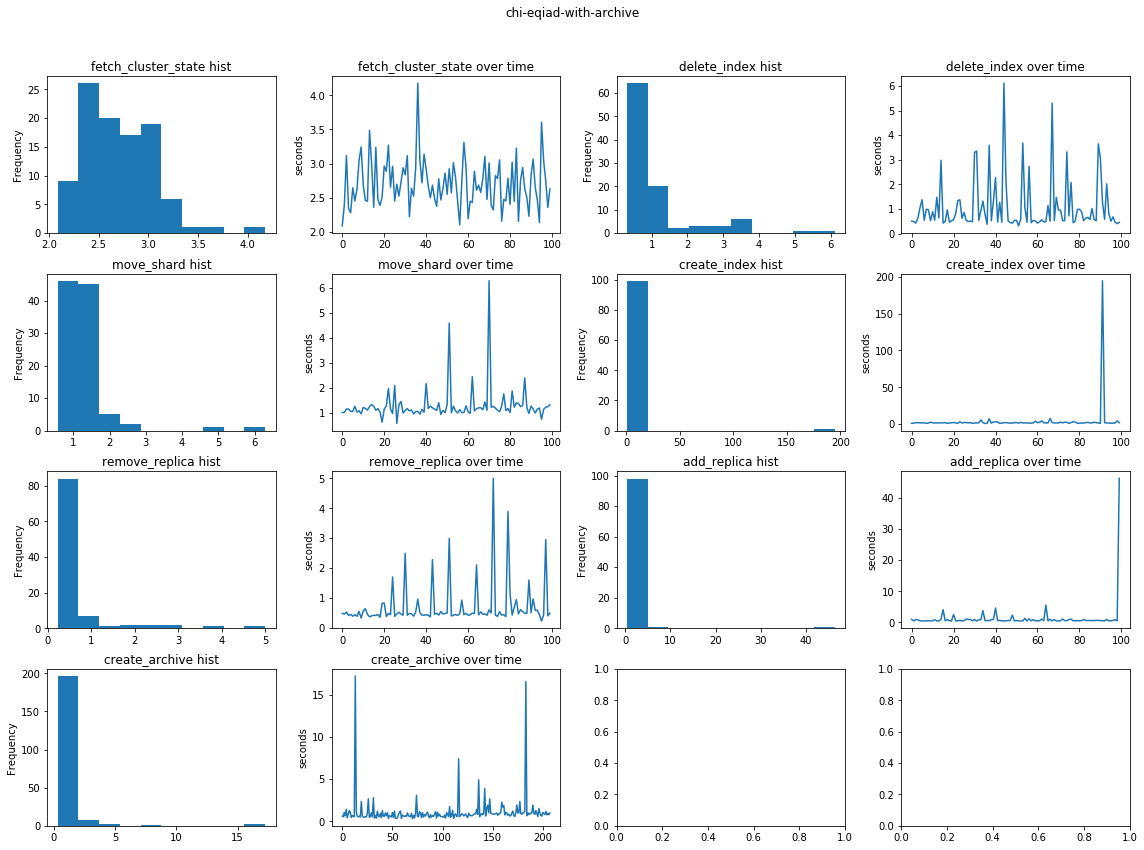In :
import math
import matplotlib.pyplot as plt
import numpy as np
import pandas as pd

with open(path, 'r') as f:
for line in f:
pieces = line.strip().split(':')
if len(pieces) == 2:
metric, value = pieces
elif len(pieces) == 3:
timestamp, metric, value = pieces
else:
raise Exception("Couldn't parse line: %s" % (line))
yield ident, metric, float(value)

def inner():
for ident, path in paths:
df = pd.DataFrame(inner())
df.columns = ('treatment', 'metric', 'value')
return df

def reject_outliers(df_in, k=3):
# k is the number of allowed standard deviations away from the mean
aggs = df_in.groupby(['treatment', 'metric']).aggregate([np.mean, np.std])
max_val = aggs['value']['mean'] + (k * aggs['value']['std'])
max_val.name = 'max_val'
min_val = aggs['value']['mean'] - (k * aggs['value']['std'])
min_val.name = 'min_val'
df_out = df_in.join(min_val, on=['treatment', 'metric']).join(max_val, on=['treatment', 'metric'])
condition = (df_out['min_val'] < df_out['value']) & (df_out['value'] < df_out['max_val'])
return df_out[condition][['treatment', 'metric', 'value']]

def report(path_format, n_indices):
df = read_all_metrics((index_count, path_format.format(index_count)) for index_count in n_indices)
df_agg = df.groupby(['metric', 'treatment']).mean().reset_index() \
.pivot(index='treatment', columns='metric', values='value')
# Data collection was bad and included fetch_cluster_state in move_shard collection
df_agg['move_shard'] -= df_agg['fetch_cluster_state']
# Not meaningful like this
del df_agg['dupe']
ax = df_agg.plot(xlim=(0,2800), ylim=(0, 8))
ax.set_ylabel('seconds')

def compare(*paths, max_std=None):
df = read_all_metrics((x, x) for x in paths)
if max_std is not None:
df = reject_outliers(df, max_std)
df_agg = (df[df['metric'] != 'dupe']
.groupby(['treatment', 'metric'])
.aggregate([np.min, np.mean, np.max, np.std])
.unstack('metric').transpose()
.reset_index(level=0, drop=True)
.rename_axis(['aggregation', 'metric'])
.reset_index()
.sort_values(['metric', 'aggregation'])
.set_index(['metric', 'aggregation']))
return df_agg

def detail_report(path):
metrics = list(df['metric'].unique())
if 'create_archive' in metrics:
metrics.remove('create_archive')
metrics.append('create_archive')
height = int(math.ceil(len(metrics)/2.0))
fig, axes = plt.subplots(height, 4, figsize=(16, 3 * height))
fig.suptitle(path)
for i, metric in enumerate(metrics):
x = i // 2
y = 0 if i % 2 == 0  else 2
df_metric = df[df['metric'] == metric].reset_index(drop=True)
axes[x][y].set_title('{} hist'.format(metric))
df_metric.value.plot.hist(ax=axes[x][y])
axes[x][y + 1].set_title('{} over time'.format(metric))
axes[x][y + 1].set_ylabel('seconds')
df_metric.value.plot(ax=axes[x][y + 1])
plt.tight_layout()


## 4 node cluster on laptop¶

These first two graphs are from a 4 node cluster running on a laptop to check initial feasability and develop measurement software. They y axis is the mean number of seconds to complete an operation. The x axis is the number of non-archive indices that exist. The first graph has, in addition to the specified number of indices, an expected (1/3 of index count) number of additional archive indices that we are measuring the impact of adding.

In :
report('latencies-archive-{}', (10, 30, 90, 270, 810, 1620, 2798))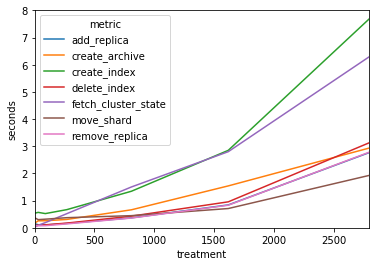In :
report('latencies-{}', (10, 30, 90, 270, 810, 1620))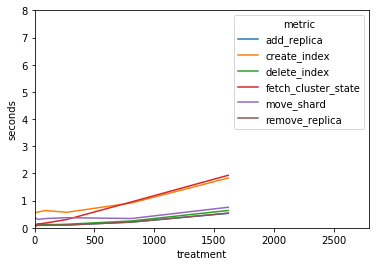## Compare production clusters¶

Compare latencies of various operations on the clusters under different treatments. codfw tests run from wasat. eqiad tests run from terbium. Minor changes in the data collection were made between runs. all values reported here are in seconds.

Race conditions (and sub-par data collection) mean that sometimes actions that cause the cluster to go green->yellow, such as adding a replica, are recorded into the next action that waits for green.

In :
from IPython.display import display

reports = ['{}-{}{}'.format(cluster, dc, suffix)
for cluster in ('psi', 'chi', 'omega')
for suffix in ('', '-with-archive')]
for cluster in ('psi', 'chi', 'omega'):
display(compare(*('{}-{}{}'.format(cluster, dc, suffix) for suffix in ('', '-with-archive'))))

metric aggregation
amin 0.284007 0.412462
mean 0.453486 0.845574
std 0.173732 0.442530
create_archive amax NaN 9.733179
amin NaN 0.676889
mean NaN 1.181620
std NaN 0.980406
create_index amax 1.748412 36.475646
amin 0.798888 0.981279
mean 1.055407 2.470375
std 0.214126 4.307091
delete_index amax 2.353573 2.818253
amin 0.260263 0.365700
mean 0.541047 0.988521
std 0.350684 0.583953
fetch_cluster_state amax 4.362320 4.819047
amin 2.300280 2.918922
mean 3.135959 3.785449
std 0.366079 0.423175
move_shard amax 1.651968 4.231689
amin 0.378666 0.935276
mean 0.939027 1.488404
std 0.185134 0.476390
remove_replica amax 0.686313 2.077054
amin 0.266160 0.363733
mean 0.376752 0.733203
std 0.094806 0.341195
metric aggregation
amin 0.485043 0.409097
mean 1.009359 1.284418
std 0.469344 4.626562
create_archive amax NaN 17.250039
amin NaN 0.314673
mean NaN 1.090191
std NaN 1.723468
create_index amax 63.110431 194.680247
amin 0.980831 0.846730
mean 2.711586 3.913923
std 6.131714 19.300513
delete_index amax 6.682615 6.113952
amin 0.253268 0.310206
mean 1.444515 1.083327
std 1.049900 1.066920
fetch_cluster_state amax 2.722839 4.176132
amin 1.641279 2.088655
mean 2.127756 2.707942
std 0.268448 0.350443
move_shard amax 4.061344 6.289596
amin 0.781141 0.569589
mean 1.346381 1.280889
std 0.488110 0.676869
remove_replica amax 9.191319 5.000494
amin 0.240559 0.228756
mean 0.984504 0.703113
std 1.110422 0.739870
metric aggregation
amin 0.389913 0.454293
mean 0.559073 0.817754
std 0.207199 0.524519
create_archive amax NaN 15.464149
amin NaN 0.600245
mean NaN 1.165978
std NaN 1.033703
create_index amax 2.403932 291.071260
amin 0.742246 1.021956
mean 1.265074 4.681635
std 0.413879 28.974827
delete_index amax 1.263980 6.491376
amin 0.329946 0.446455
mean 0.505980 0.882809
std 0.207916 0.816100
fetch_cluster_state amax 4.089278 4.843013
amin 2.650902 3.095127
mean 3.203850 3.996883
std 0.377167 0.454029
move_shard amax 1.241029 1.711373
amin 0.517315 1.323078
mean 1.067055 1.483154
std 0.121189 0.124994
remove_replica amax 1.596538 4.506533
amin 0.331686 0.438639
mean 0.463270 0.720094
std 0.196985 0.642949
treatment psi-codfw psi-codfw-with-archive
metric aggregation
amin 0.511079 0.553371
mean 1.022711 1.164675
std 0.274513 0.263898
create_archive amax NaN 1.514391
amin NaN 0.440393
mean NaN 0.648939
std NaN 0.113343
create_index amax 1.815361 1.858168
amin 0.836244 0.924959
mean 1.266745 1.346399
std 0.185950 0.164690
delete_index amax 0.747143 0.625578
amin 0.320923 0.351336
mean 0.401220 0.388818
std 0.076002 0.037694
fetch_cluster_state amax 4.695016 4.094146
amin 2.377671 2.774941
mean 3.265336 3.361268
std 0.573914 0.306230
move_shard amax 1.259534 1.374253
amin 0.452415 0.530548
mean 0.925975 0.994980
std 0.207508 0.183639
remove_replica amax 0.553617 0.693972
amin 0.307576 0.348142
mean 0.359248 0.376709
std 0.030037 0.045333
treatment chi-codfw chi-codfw-with-archive
metric aggregation
amin 0.482686 0.524209
mean 0.840587 0.791842
std 0.245589 0.116137
create_archive amax NaN 11.423560
amin NaN 0.404857
mean NaN 0.707950
std NaN 0.779678
create_index amax 14.293614 2.857695
amin 0.767582 0.777848
mean 1.146992 0.930634
std 1.353164 0.304025
delete_index amax 1.049806 0.710945
amin 0.305940 0.326263
mean 0.392095 0.387373
std 0.109561 0.068775
fetch_cluster_state amax 3.319163 2.898446
amin 1.762060 1.970118
mean 2.216643 2.370863
std 0.416274 0.223110
move_shard amax 3.261147 1.028186
amin 0.559117 0.497986
mean 0.821998 0.824132
std 0.315121 0.118986
remove_replica amax 1.466925 1.405399
amin 0.299062 0.316189
mean 0.377101 0.373123
std 0.125765 0.131555
treatment omega-codfw omega-codfw-with-archive
metric aggregation
amin 0.494155 0.541910
mean 0.833955 0.957839
std 0.219049 0.197405
create_archive amax NaN 10.715171
amin NaN 0.448563
mean NaN 0.710282
std NaN 0.683334
create_index amax 12.130562 1.417956
amin 0.738262 0.875968
mean 1.065575 0.991173
std 1.135723 0.146910
delete_index amax 0.906493 0.889648
amin 0.304015 0.340288
mean 0.378573 0.389599
std 0.100293 0.074852
fetch_cluster_state amax 4.802250 5.298962
amin 2.723684 2.995567
mean 3.470338 3.768098
std 0.680308 0.541050
move_shard amax 1.640414 1.418294
amin 0.431839 0.539606
mean 0.918518 1.053511
std 0.211874 0.190068
remove_replica amax 0.845920 0.426370
amin 0.294826 0.336764
mean 0.346358 0.362251
std 0.101348 0.015353

## Detailed per-run reports¶

The rest of the graphs here show details about test runs in terms of a histogram and an over-time graph per metric

In :
for report in reports:
detail_report(report)